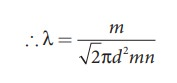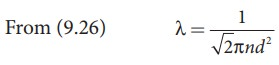Home | | Physics 11th std | Mean Free Path

# Mean Free Path

This average distance travelled by the molecule between collisions is called mean free path (λ). We can calculate the mean free path based on kinetic theory.

MEAN FREE PATH

Usually the average speed of gas molecules is several hundred meters per second even at room temperature. Odor from an open perfume bottle takes some time to reach us even if we are closer to the room. The time delay is because the odor of the molecules cannot travel straight to us as it undergoes a lot of collisions with the nearby air molecules and moves in a zigzag path. This average distance travelled by the molecule between collisions is called mean free path (λ). We can calculate the mean free path based on kinetic theory.

## Expression for mean free path

We know from postulates of kinetic theory that the molecules of a gas are in random motion and they collide with each other. Between two successive collisions, a molecule moves along a straight path with uniform velocity. This path is called mean free path.

Consider a system of molecules each with diameter d. Let n be the number of molecules per unit volume.

Assume that only one molecule is in motion and all others are at rest as shown in the Figure 9.8If a molecule moves with average speed v in a time t, the distance travelled is vt. In this time t, consider the molecule to move in an imaginary cylinder of volume πd2vt. It collides with any molecule whose center is within this cylinder. Therefore, the number of collisions is equal to the number of molecules in the volume of the imaginary cylinder. It is equal to πd2vtn. The total path length divided by the number of collisions in time t is the mean free path.Though we have assumed that only one molecule is moving at a time and other molecules are at rest, in actual practice all the molecules are in random motion. So the average relative speed of one molecule with respect to other molecules has to be taken into account. After some detailed calculations (you will learn in higher classes) the correct expression for mean free pathThe equation (9.26) implies that the mean free path is inversely proportional to number density. When the number density increases the molecular collisions increases so it decreases the distance travelled by the molecule before collisions.

Case1: Rearranging the equation (9.26) using ‘m’ (mass of the molecule)But mn=mass per unit volume = ρ (density of the gas)The equation (9.28) implies the following

1. Mean free path increases with increasing temperature. As the temperature increases, the average speed of each molecule will increase. It is the reason why the smell of hot sizzling food reaches several meter away than smell of cold food.

2. Mean free path increases with decreasing pressure of the gas and diameter of the gas molecules.

### EXAMPLE 9.6

An oxygen molecule is travelling in air at 300 K and 1 atm, and the diameter of oxygen molecule is 1.2 × 10−10m. Calculate the mean free path of oxygen molecule.

### SolutionWe have to find the number density n By using ideal gas lawTags : Kinetic Theory of Gases | Physics , 11th Physics : UNIT 9 : Kinetic Theory of Gases
Study Material, Lecturing Notes, Assignment, Reference, Wiki description explanation, brief detail
11th Physics : UNIT 9 : Kinetic Theory of Gases : Mean Free Path | Kinetic Theory of Gases | Physics